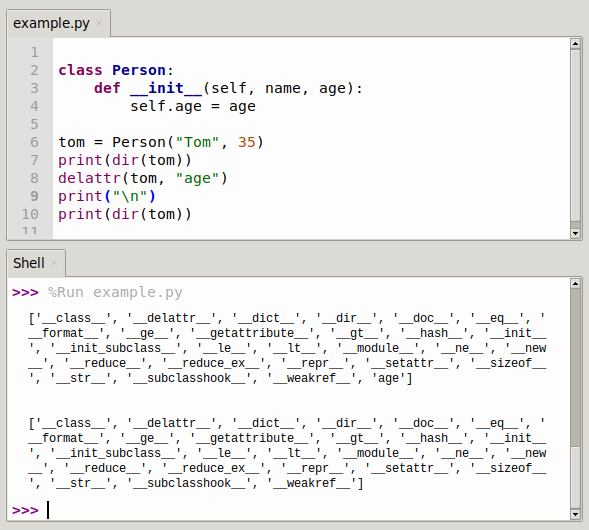# Python delattr function

The `delattr(object, name)` remove the name attribute from the object. The purpose is to delete an attribute.

An object (derived from a class) often has one more attributes, Python allows you to delete one or more of those attributes while the program is running.

## experimental code

The code below shows the deletion of an attribute and requesting the objects data.

``````class Person:
def __init__(self, name, age):
class Person(self, name, age):
self.name = name
self.age = age

tom = Person("Tom", 35)
print(dir(tom))
delattr(tom, "age")
print(dir(tom))``````

Result:

``````['__doc__', '__init__', '__module__', 'age', 'name']
['__doc__', '__init__', '__module__', 'name']``````

## How to Use the Python delattr() Function?

The following example shows how to use the delattr() function

``````class Coordinate:
x = 11
y = -6
z = 1

point1 = Coordinate()
print('x = ', point1.x)
print('y = ', point1.y)
print('z = ', point1.z)

delattr(Coordinate, 'z')
print('--after deleting the z property--')
print('x = ', point1.x)
print('y = ', point1.y)

# Trigger error
print('property deleted z = ',point1.z)``````

Output

``````x = 11
y = -6
z = 1
--- After deleting the z property--
x = 11
y = -6
Traceback (most recent call last):
File "D:/Pythonproject/111/delattr.py", line 14, in <module>
print('attribute deleted z = ',point1.z)
AttributeError: 'Coordinate' object has no attribute 'z'``````

This is where the delattr() function “learns”.## Deleting attribute with Python del operator

An alternative to the delattr() method is calling the del operator. Both do exactly the same: delete an attribute.

``````>>> class A:
...     x = 1
...     y = 2
...     z = 3
...
>>> print(A.z)
3

# delete attribute
>>> del A.z

# try getting value
>>> print(A.z)
Traceback (most recent call last):
File "<stdin>", line 1, in <module>
AttributeError: type object 'A' has no attribute 'z'
>>> ``````

## Python delattr() method v/s Python del operator

The `delattr()` function is more efficient that the del operator for deletion of the attributes. But the del operator is faster than calling the delattr() method.